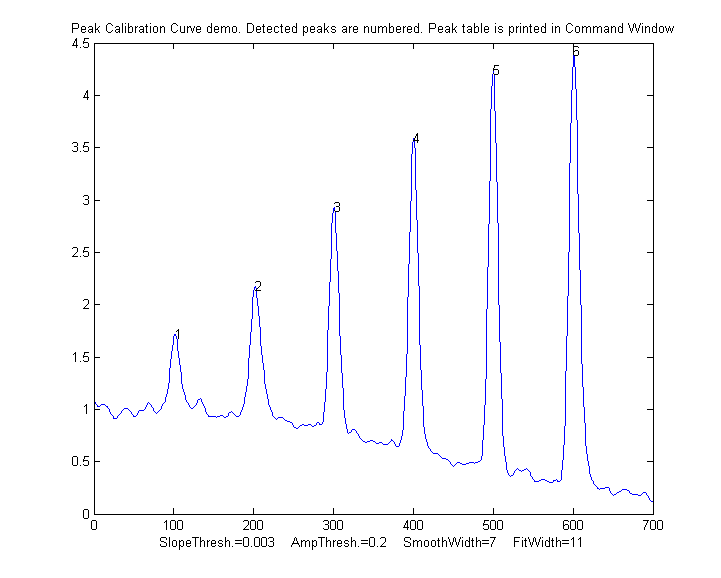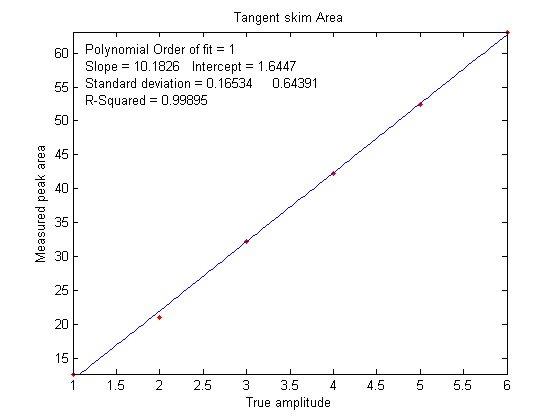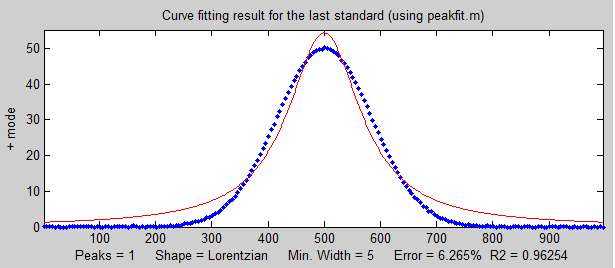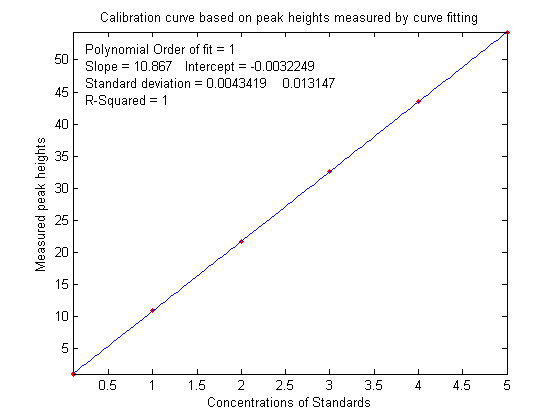## Appendix U. Measurement Calibration

Most scientific measurements involve the use of an instrument that actually measures something else and converts it to the desired measure. Examples are simple weight scales (which actually measure the compression of a spring), thermometers (which actually measure thermal expansion), pH meters (which actually measure a voltage), and devices for measuring hemoglobin in blood or CO2 in air (which actually measure the intensity of a light beam). These instruments are single-purpose, designed to measure one quantity, and automatically convert what they actually measure into the the desired quantity and display it directly. But to insure accuracy, such instruments must be calibrated, that is, used to measure one or more calibration standards of known accuracy, such as a standard weight or a sample that is carefully prepared to a known temperature, pH, or sugar content. Most are pre-calibrated at the factory for the measurement of a specific substance in a specific type of sample.

Analytical calibration. General-purpose instrumental techniques that are used to measure the quantity of many different chemical components in unknown samples, such as the various kinds of spectroscopy, chromatography, and electrochemistry, or combination techniques like "GC-mass spec", must also be calibrated, but because those instruments can be used to measure a wide range of compounds or elements, they must be calibrated by the user for each substance and for each type of sample. Usually this is accomplished by carefully preparing (or purchasing) one or more "standard samples" of known concentration, such as solution samples in a suitable solvent. Each standard is inserted or injected into the instrument, and the resulting instrument readings are plotted against the known concentrations of the standards, using least-squares calculations to compute the slope and intercept, as well as the standard deviation of the slope (sds) and intercept (sdi). Then the "unknowns" (that is, the samples whose concentrations are to be determined) are measured by the instrument and their signals are converted into concentrations with the aid of the calibration curve. If the calibration is linear, the sample concentration C of any unknown is given by (A - intercept) / slope, where A is the measured signal (height or area) of that unknown. The predicted standard deviation in the sample concentration is C*SQRT((sdi/(A-intercept))^2+(sds/slope)^2) by the rules for propagation of error. All these calculations can be done in a spreadsheet, such as CalibrationLinear.xls.

In some cases the thing measured can not be detected directly but must undergo a chemical reaction that makes it measurable; in that case the exact same reaction must be carried out on all the standard solutions and unknown sample solutions, as demonstrated in this animation (thanks to Cecilia Yu of Wellesley College).

Various calibration methods are used to compensate for problems such as random errors in standard preparation or instrument readings, interferences, drift, and non-linearity in the relationship between concentration and instrument reading. For example, the standard addition calibration technique can be used to compensate for multiplicative interferences. I have prepared a series of "fill-in-the-blanks" spreadsheet templates for various calibrations methods, with instructions, as well as a series of spreadsheet-based simulations of the error propagation in widely-used analytical calibration methods, including a step-by-step exercise.

Calibration and signal processing. Signal processing often intersects with calibration. For example, if you use smoothing or filtering to reduce noise, or differentiation to reduce the effect of background, or measure peak area to reduce the effect of peak broadening, or use modulation to reduce the effectof low-frequency drift, then you must use the exact same signal processing for both the standard samples and the unknowns, because the choice of signal processing technique can have a big impact on the magnitude and even on the units of the resulting processed signal (as for example in the derivative technique and in choosing between peak height and peak area).

PeakCalibrationCurve.m is an Matlab/Octave example of this. This script simulates the calibration of a flow injection system that produces signal peaks that are related to an underlying concentration or amplitude ('amp'). In this example, six known standards are measured sequentially, resulting in six separate peaks in the observed signal. (We assume that the detector signal is linearly proportional to the concentration at any instant). To simulate a more realistic measurement, the script adds four sources of "disturbance" to the observed signal:

a. noise - random white noise added to all the signal data points, controlled by the variable "Noise";
b. background - broad curved background of random amplitude, tilt, and curvature, controlled by "background";
d. a final smoothing before the peaks are measured, controlled by "FinalSmooth".

The script uses measurepeaks.m as an internal function to determine the absolute peak height, peak-valley difference, perpendicular drop area, and tangent skim area. It plots separate calibration curve for each of these measures in figure windows 2-5 against the true underlying amplitudes (in the vector "amp"), fitting the data to a straight line and computing the slope, intercept, and R2. (If the detector response were non-linear, a quadratic or cubic least-square would work better). The slope and intercept of the best-fit line is different for the different methods, but if the R2 is close to 1.000, a successful measurement can be made. (If all the random disturbances are set to zero in lines 33-36, the R2 values will all be 1.000. Otherwise the measurements will not be perfect and some methods will result in better measurements - R2 closer to 1.000 - than others). Here is a typical result:Peak Position PeakMax Peak-val. Perp drop Tan skim
1    101.56   1.7151  0.72679   55.827    11.336
2    202.08   2.1775  1.2555    66.521    21.425
3    300.7    2.9248  2.0999    58.455    29.792
4    400.2    3.5912  2.949     66.291    41.264
5    499.98   4.2366  3.7884    68.925    52.459
6    601.07   4.415   4.0797    75.255    61.762
R2 values:        0.9809  0.98615   0.7156    0.99824

In this case, the tangent skim method works best, giving a linear calibration curve (shown on the left) with the highest R2.

In this type of application, the peak heights and/or area measurements do not actually have to be
accurate, but they must be precise. That's because the objective of an analytical method such as flow injection or chromatography is not to measure peak heights and areas, but rather to measure concentrations, which is why calibration curves are used. Figure 6 shows the correlation between the measured tangent skim areas and the actual true areas under the peaks in the signal shown above, right; the slope of this plot shows that the tangent skim areas are actually about 6% lower that the true areas, but that does not make a difference in this case because the standards and the unknown samples are measured the same way. In some other application, you may actually need to measure the peak heights and/or areas accurately, in which case curve fitting is generally the best way to go.

If the peaks partly overlap, the measured peak heights and areas may be effected. To reduce the problem, it may be possible to reduce the overlap by using peak sharpening methods, for example the derivative method, deconvolution or the power transform method, as demonstrated by the self-contained Matlab/Octave function PowerTransformCalibrationCurve.m.

Curve fitting the signal data. Ordinary in curve fitting, such as the classical least squares (CLS) method and in iterative nonlinear least-squares, the selection of a model shape is very important. But in quantitative analysis applications of curve fitting, where the peak height or area measured by curve fitting is used only to determine the concentration of the substance that created the peak by constructing a calibration curve, having the exact model shape is surprisingly uncritical. The Matlab/Octave script PeakShapeAnalyticalCurve.m shows that, for a single isolated peak whose shape is constant and independent of concentration, if the wrong model shape is used, the peak heights measured by curve fitting will be inaccurate, but that error will be exactly the same for the unknown samples and the known calibration standards, so the error will "cancel out" and the measured concentrations will still be accurate, provided you use the same inaccurate model for both the known standards and the unknown samples.In the example shown on the right, the peak shape of the actual peak is Gaussian (blue dots) but the model used to fit the data is Lorentzian (red line). That's an intentionally bad fit to the signal data; the R2 value for the fit to the signal data is only 0.962 (a poor fit by the standards of measurement science). The result of this is that the slope of the calibration curve (shown below on the left) is greater than expected; it should have been 10 (because that's the value of the "sensitivity" in line 18), but it's actually 10.867 in the figure on the left, but nevertheless the calibration curve is still linear and its R2 value is 1.000, meaning that the analysis should be accurate. (Note that curve fitting is actually applied twice in this type of application, once using iterative curve fitting to fit the signal data, and then again using polynomial curve fitting to fit the calibration data).

Despite all this, it's still better to use as accurate a model peak shape as possible for the signal data, because the percent fitting error of the signal fit can be used as a warning that something unexpected is wrong, such as the appearance of an interfering peak from a foreign substance.

This page is part of "A Pragmatic Introduction to Signal Processing", created and maintained by Prof. Tom O'Haver , Department of Chemistry and Biochemistry, The University of Maryland at College Park. Comments, suggestions and questions should be directed to Prof. O'Haver at toh@umd.edu. Updated July, 2022.